# Important Questions Class 12 Maths Chapter 11-Three Dimensional Geometry

Important Questions For Class 12 Maths Chapter 11 Three Dimensional Geometry are available here to help the students who are appearing for the CBSE board exams. All the concepts of Three Dimensional Geometry for 12th standard are important for students from the examination point of view to get more marks.

Students can refer to all the important problems and solutions for different concepts provided at BYJU’S for better preparation of exams. Also, they can access the solutions for all exercise questions in NCERT book of Class 12 Maths with detailed explanations. For important questions of 12th maths, all chapters reach us at BYJU’S.

## Important Questions & Answers for Class 12 Maths Chapter 11 Three Dimensional Geometry

Q. 1: Find the direction cosines of the line passing through the two points (– 2, 4, – 5) and (1, 2, 3).

Solution:

We know that the direction cosines of the line passing through two points P(x1, y1, z1) and Q(x2, y2, z2) are given by

$$\begin{array}{l}\frac{x_2-x_1}{PQ}, \frac{y_2-y_1}{PQ}, \frac{z_2-z_1}{PQ}\end{array}$$

Using the distance formula,

$$\begin{array}{l}PQ=\sqrt{(x_2-x_1)^2+(y_2-y_1)^2+(z_2-z_1)^2}\end{array}$$

From the given,

P(x1, y1, z1) = (-2, 4, -5) and Q(x2, y2, z2) = (1, 2, 3)

$$\begin{array}{l}PQ=\sqrt{(1-(-2))^2+(2-4)^2+(3-(-5))^2}\end{array}$$
$$\begin{array}{l}PQ=\sqrt{9+4+64}\end{array}$$
$$\begin{array}{l}PQ=\sqrt{77}\end{array}$$

Hence, the direction cosines of the line joining the given two points are

$$\begin{array}{l}\frac{3}{\sqrt{77}}, \frac{-2}{\sqrt{77}}, \frac{8}{\sqrt{77}}\end{array}$$

Q. 2: Show that the points A (2, 3, – 4), B (1, – 2, 3) and C (3, 8, – 11) are collinear.

Solution:

We know that the direction ratios of the line passing through two points P(x1, y1, z1) and Q(x2, y2, z2) are given by:

x2 – x1, y2 – y1, z2 – z1 or x1 – x2, y1 – y2, z1 – z2

Given points are A (2, 3, – 4), B (1, – 2, 3) and C (3, 8, – 11).

Direction ratios of the line joining A and B are:

1 – 2, – 2 – 3, 3 + 4

i.e. – 1, – 5, 7.

The direction ratios of the line joining B and C are:

3 –1, 8 + 2, – 11 – 3

i.e., 2, 10, – 14.

From the above, it is clear that direction ratios of AB and BC are proportional.

That means AB is parallel to BC. But point B is common to both AB and BC.

Hence, A, B, C are collinear points.

Q. 3: If a line makes angles 90°, 135°, 45° with the x, y and z-axes respectively, find its direction cosines.

Solution:

Let the direction cosines of the line be l, m, and n.

l = cos 90° = 0

m = cos 135° = -1/√2

n = cos 45° = 1/√2

Hence, the direction cosines of the line are 0, -1/√2, and 1/√2.

Q. 4: Find the angle between the pair of lines given by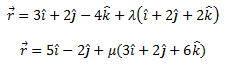Solution:

From the given,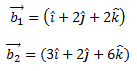Let θ be the angle between the given pair of lines.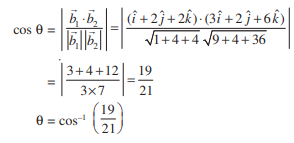Q. 5: Find the angle between the pair of lines given below.

(x + 3)/3 = (y -1)/5 = (z + 3)/4

(x + 1)/1 = (y – 4)/1 = (z – 5)/2

Solution:

Given,

(x + 3)/3 = (y -1)/5 = (z + 3)/4

(x + 1)/1 = (y – 4)/1 = (z – 5)/2

The direction ratios of the first line are:

a1 = 3, b1 = 5, c1 = 4

The direction ratios of the second line are:

a2 = 1, b2 = 1, c2 = 2

$$\begin{array}{l}cos\ \theta =\left | \frac{a_1a_2+b_1b_2+c_1c_2}{\sqrt{a_1^2+b_1^2+c_1^2}{\sqrt{a_2^2+b_2^2+c_2^2}}} \right |\end{array}$$
$$\begin{array}{l}cos\ \theta =\left | \frac{3.1+5.1+4.2}{\sqrt{3^2+5^2+4^2}{\sqrt{1^2+1^2+2^2}}} \right |\end{array}$$
$$\begin{array}{l}=\frac{16}{\sqrt{50}\sqrt{6}}\end{array}$$
$$\begin{array}{l}=\frac{16}{5\sqrt{2}\sqrt{6}}\end{array}$$
$$\begin{array}{l}=\frac{8\sqrt{3}}{15}\end{array}$$

Hence, the required angle is

$$\begin{array}{l}\theta=cos^{-1}(\frac{8\sqrt{3}}{15})\end{array}$$

Q. 6: Find the distance between the lines l1 and l2 given by: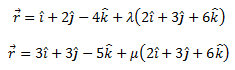Solution:

Given two lines are parallel.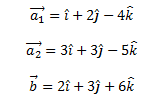The distance between the two given lines is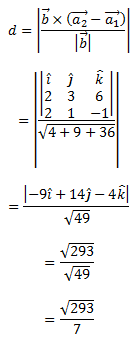Q. 7: Show that the lines (x – 5)/7 = (y + 2)/-5 = z/1 and x/1 = y/2 = z/3 are perpendicular to each other.

Solution:

Given lines are:

(x – 5)/7 = (y + 2)/-5 = z/1 and x/1 = y/2 = z/3

The direction ratios of the given lines are 7, -5, 1 and 1, 2, 3, respectively.

We know that,

Two lines with direction ratios a1, b1, c1 and a2, b2, c2 are perpendicular to each other if a1a2 + b1b2 + c1c2 = 0

Therefore, 7(1) + (-5) (2) + 1 (3)

= 7 – 10 + 3

=0

Hence, the given lines are perpendicular to each other.

$$\begin{array}{l}\text{Q. 8: Find the vector equation of a plane which is at a distance of 7 units from the origin and} \\ \text{normal to the vector } 3\hat{i}+5\hat{j}-6\hat{k}.\end{array}$$

Solution:

Given, the normal vector is: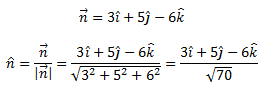$$\begin{array}{l}\text{We know that the equation of the plane with position vector } \vec{r} \text{ is given by }\end{array}$$
$$\begin{array}{l}\vec{r}.\hat{n}=d\end{array}$$

Hence, the vector equation of the required plane is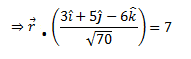Q. 9: Find the intercepts cut off by the plane 2x + y – z = 5.

Solution:

Given plane is 2x + y – z = 5 ……(i)

Dividing both sides of the equation (i) by 5,

(⅖)x + (y/5) – (z/5) = 1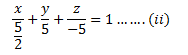We know that,

The equation of a plane in intercept form is (x/a) + (y/b) + (z/c) = 1, where a, b, c are intercepts cut off by the plane at x, y, z-axes respectively.

For the given equation,

a = 5/2, b = 5, c = -5

Hence, the intercepts cut off by the plane are 5/2, 5 and -5.

Q. 10: Find the equations of the planes that passes through three points (1, 1, 0), (1, 2, 1), and (– 2, 2, – 1).

Solution:

Given points are (1, 1, 0), (1, 2, 1), and (– 2, 2, – 1).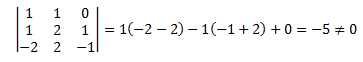Therefore, the plane will pass through the given three points.

We know that,

The equation of the plane through the points (x1, y1, z1), (x2, y2, z2) and (x3, y3, z3) is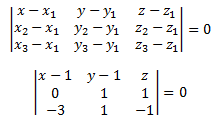(x – 1)(-2) -(y – 1) (3) + z (3) = 0

-2x + 2 – 3y + 3 + 3z = 0

-2x – 3y + 3z + 5 = 0

-2x – 3y + 3z = -5

Therefore, 2x + 3y – 3z = 5 is the required Cartesian equation of the plane.

### Practice Questions For Class 12 Maths Chapter 11 Three Dimensional Geometry

1. Find the direction cosines of a line whose direction ratios are 2, -6, 3. (Answer: 7)
2. Find the direction cosines of a line that makes equal angles with the coordinate axes. (Answer: 1/√3, 1/√3, 1/√3)
3. Find the angles of triangle ABC whose vertices are A(-1, 3, 2), B (2, 3, 5) and C(3, 5, -2). (Answer: A = pi/2, B =cos-1(1/√3), C =cos-1(√2/5)
4. Find the angles between the lines whose direction ratios are 3, 2, -6 and 1, 2, 2. ( Answer: cos-1(5/21)
5. A line makes an angle 60 degree and 45 degrees with the positive direction of x-axis and y-axis respectively. What acute angle does it make with the z-axis? (Answer: 60 degrees)
6. Show that the lines (x-1)/2=(y-2)/2=(z-3)/2 and (x-4)/5 = (y-1)/2 = z intersect each other. Also, find the point of intersection.
7. Find the equation of the plane which is at a distance 3√3 units from origin and the normal to which is equally inclined to coordinate axis.
8. Find the angle between the lines whose direction cosines are given by the equation: l+m+n = 0, l2+m2+n2 = 0.
9. O is the origin and A is (a,b,c). Find the direction cosines of the line OA and the equation of the plane through A at the right angle to OA.